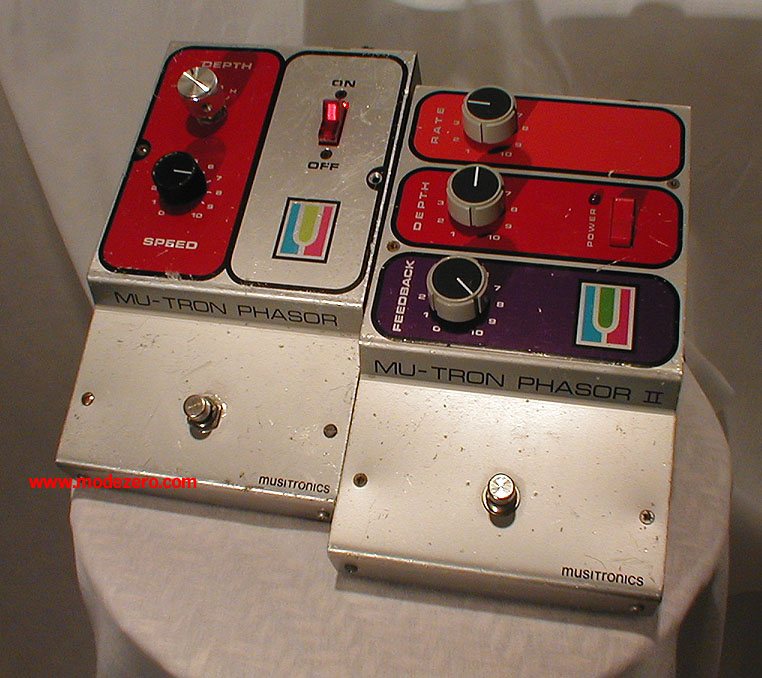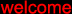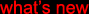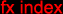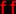(c) 2000-2009

mode zero

mu-tron phasor and phasor II

phasor: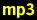matrix-1000 pad, no effectmatrix-1000 pad, depth = L, speed = 1matrix-1000 pad, depth = M, speed = 1matrix-1000 pad, depth = H, speed = 1matrix-1000 pad, depth = L, speed = 7matrix-1000 pad, depth = M, speed = 7matrix-1000 pad, depth = H, speed = 7guitar, no effectguitar, depth = H, speed = 2guitar, depth = M, speed = 8guitar, depth = H, speed = 8drum loop, no effectdrum loop, depth = H, speed = 1.5,

phasor II:prodigy saw, no effectprodigy saw, rate = 2, depth = 10, feedback = 0prodigy saw, rate = 2, depth = 10, feedback = 10prodigy saw, rate = 7, depth = 5, feedback = 0guitar, no effectguitar, rate = 5, depth = 5, feedback = 5guitar, rate = 2, depth = 10, feedback = 10guitar, rate = 7, depth = 10, feedback = 10matrix-1000 pad, no effectmatrix-1000 pad, rate = 2, depth = 10, feedback = 10matrix-1000 pad, rate = 7, depth = 10, feedback = 0matrix-1000 pad, rate = 7, depth = 10, feedback = 10jd990 brass, no effectjd990 brass, rate = 2, depth = 10, feedback = 10jd990 brass, rate = 2, depth = 10, feedback = 0drum loop, no effectdrum loop, rate = 2, depth = 7, feedback = 10drum loop, rate = 7, depth = 4, feedback = 10